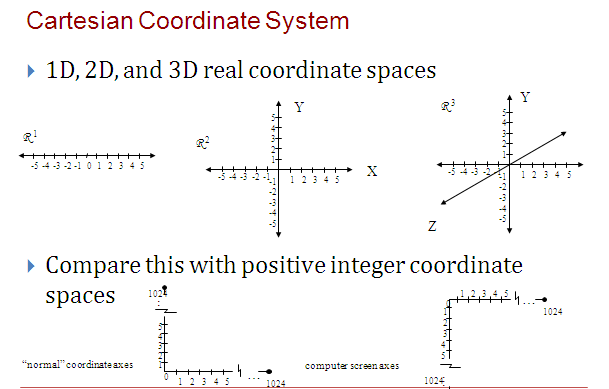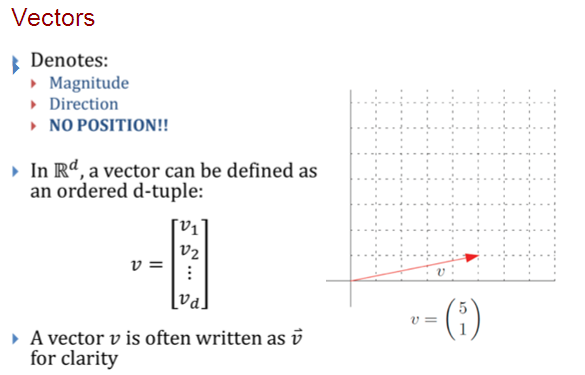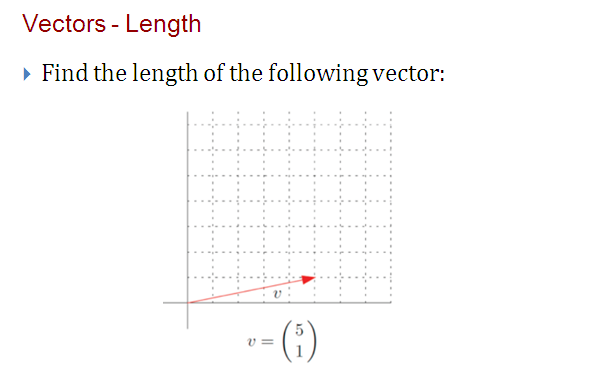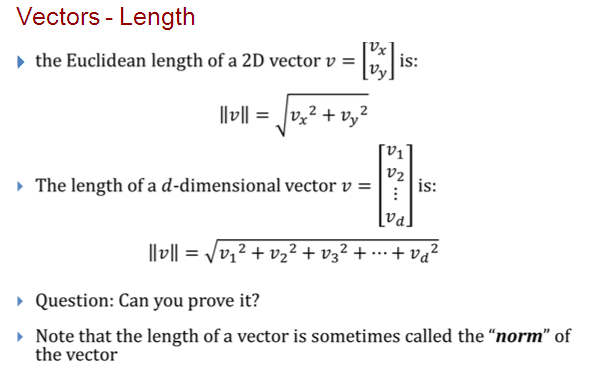# SCC Education

## Linear Algebra for 3 D

Linear Algebra for 3D
}Linear Algebra that you should know…
}3D Coordinate Geometry
}3D Points and Vectors
}dot products and cross products
}Vector and Matrix notations and algebra
}Properties of matrix multiplication (associative property,
but NOT communicative property)
}Associative => (5 + 2) + 1 = 5 + (2 + 1)
}Commutative => 5 +2 + 1 = 1 + 2 + 5
}Matrix operations (multiplication, transpose, inverse, etc.)